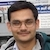# NAEST 2015 Screening Test: Filament Resistance of Electric BulbBy

In screening round of National Anveshika Experimental Skills Test ( NAEST 2015 ), a question was asked on the filament resistance of an electric bulb. The filament resistance of 100 W bulb is to be calculated. Also, the video show filament resistance measured by a multimeter. The student needs to explain the difference between calculated and measured values of filament resistance.

Question: The bulb shown in the video is a normal household bulb of 100 Watt power rating.

1. Calculate the resistance of the bulb filament?
2. How much is the difference between calculated value and measurement shown by the multi-meter? Why these two values are different?

Solution: An electric bulb has a thin tungsten filament. The power rating $P=100$ Watt is given at a rated voltage $V=220$ Volts. The calculated value of filament resistance is \begin{align} R=\frac{V^2}{P}=\frac{220\times 220}{100}=484\,\Omega. \end{align}

The multimeter reading shows a value of 41 $\Omega$. The measured value of filament resistance is very small in comparison to the calculated value. The difference is due to temperature dependance of the resistance.

The resistance of a conductor increases with temperature. When we measure the resistance of the bulb with the multimeter, the filament is at the room temperature (about 30 degree Celsius). But using the formula, the obtained resistance is the resistance of the bulb in full glow i.e., when it is connected to a 220 V and has attained a temperature at which it starts giving light. This temperature is around 3000 degree Celsius. So the calculated resistance is much higher than the resistance shown by multimeter.

The resistance of a conductor varies with temperature as \begin{align} R_T=R_0[1+\alpha(T-T_0)], \end{align} where $R_T$ is the resistance at the temperature $T$ and $R_0$ is the resistance at the temperature $T_0$ (generally the room temperature), $\alpha$ is called the temperature coefficient of resistance. The temperature coefficient of tungsten is approximately $4.5\times{10}^{-3}$ /deg C.